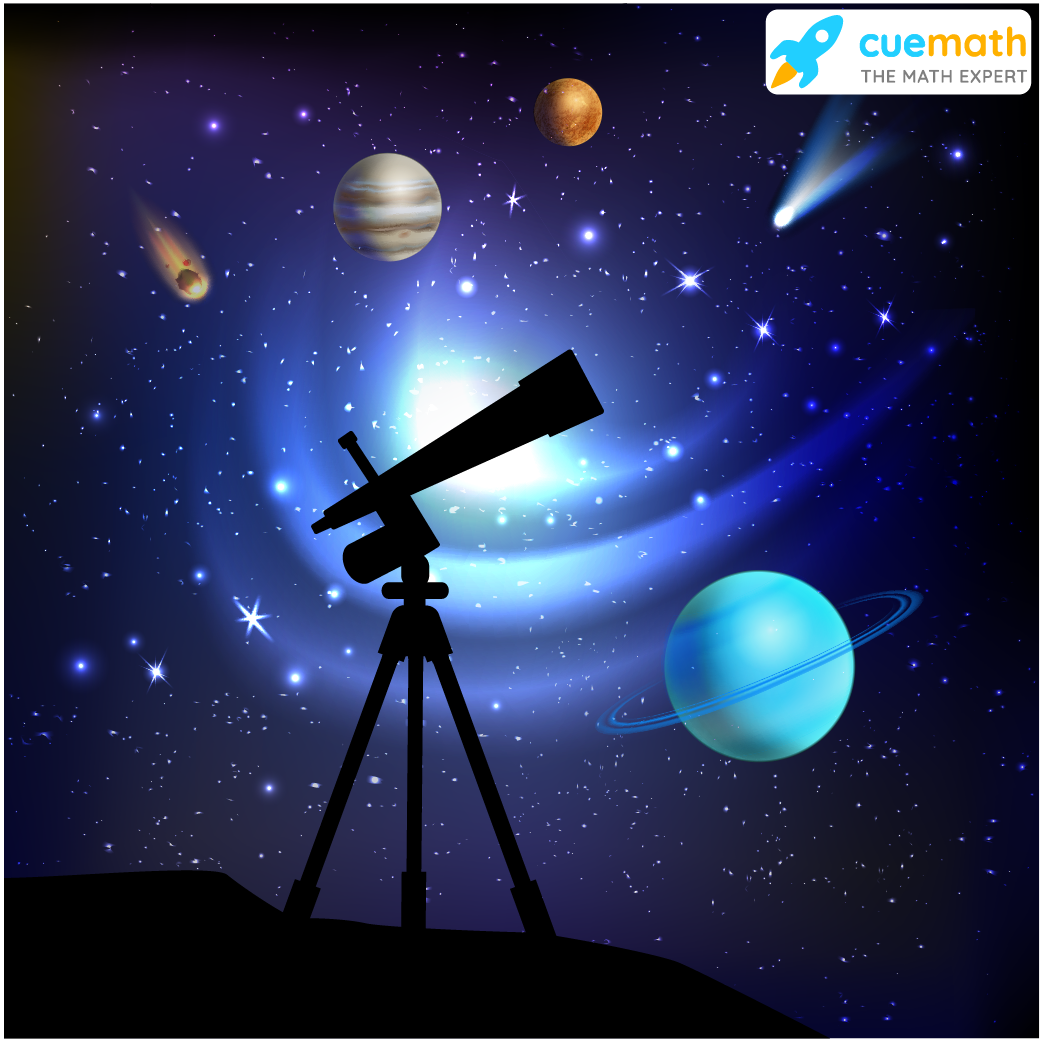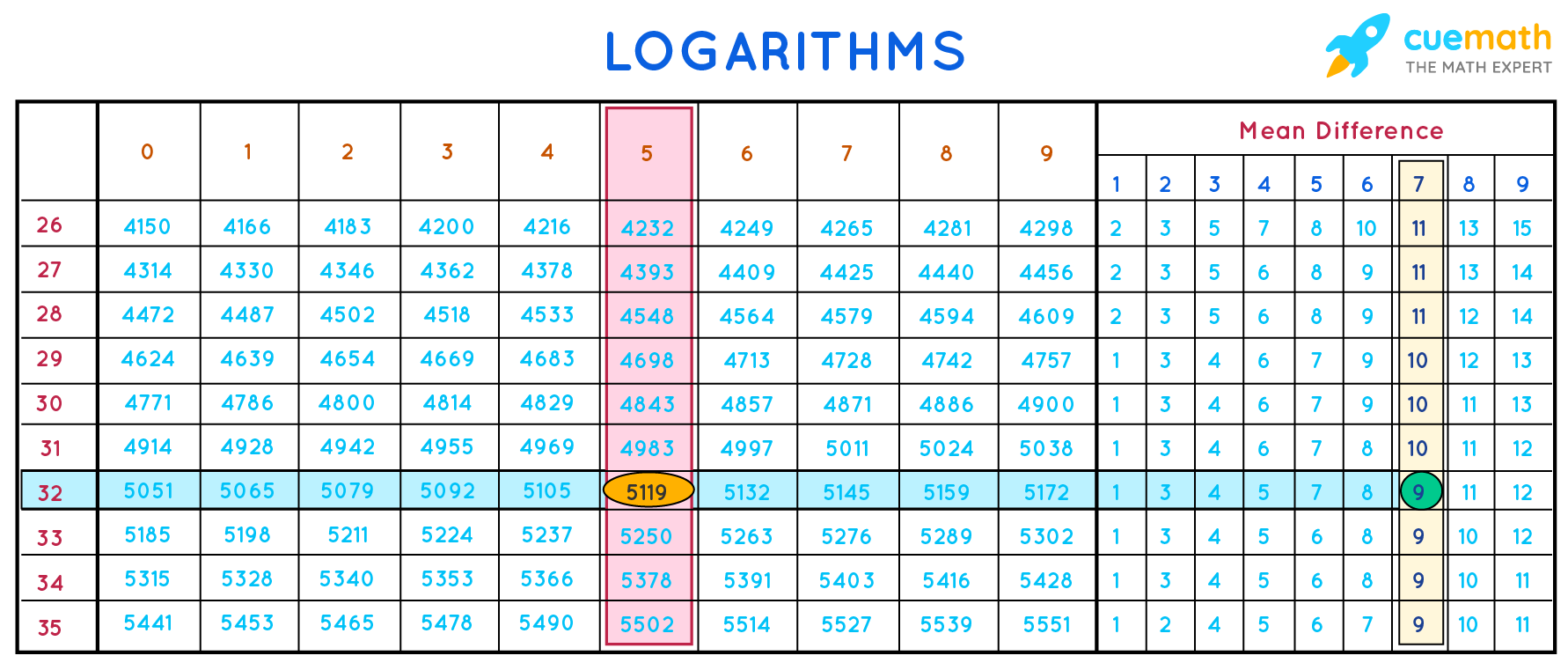# Properties of Logarithms

Properties of Logarithms

Astronomy involves calculations with large numbers such as:

\begin{align} (4.2394 \times 10^{27})^{2391}\\ 2897 \times 857^{25}\\ (0.00054316)^{43}\end{align}

In such cases, logarithms are helpful.

It does not involve the use of calculators.In this mini-lesson, we shall explore the world of logarithms by finding answers to questions like what is the meaning of log base e, what are the basic properties of logarithms, and how to find logarithms using log table.

## Lesson Plan

 1 What Do You Mean by log base e? 2 Important Notes 3 Challenging Question 4 Solved Examples on Properties of Logarithms 5 Interactive Questions on Properties of Logarithms

## What Do You Mean by log base e?

Before we begin, let us understand the log definition.

An exponential form can be converted to logarithmic form.  \begin{align} a^x &= N \\ \log_a N& = x\end{align}

In the above expression, the base of the logarithm is $$a$$.

\begin{align} 2^5 &= 32 \\ \log_2 32& = 5 \end{align}
Here the base of the logarithm is 2.

Considering the different bases, logarithms are named as common logarithms and natural logarithms.

### Natural Logarithms

As per log definition, the logarithms to the base $$e$$ are called natural logarithms. \begin{align} \log_e2 &= 0.693147 \\ e& =2.718281 \end{align}

Logarithms are also expressed to the base 10.

The logarithms to the base of 10 are called common logarithms.  $\log_{10}2 = 0.3010$

## What Are the Three Basic Properties of Logarithms?

The properties of logarithms include the following three basic log formula.

Product Rule:  $\log xy = \log x + \log y$

Division Rule:  $\log \frac{x}{y} = \log x - \log y$

Exponential Rule: $\log x^m= m\log x$

## Log Table

The log table to the base of 10 is used to find the logarithm of numbers.

These logarithms are called as common logarithms.

Let us try to understand the process of finding logarithms with the help of an example.

Find log 32.57

STEP - 1:  Look for row 32 and column 5. You will obtain the value 5119

STEP - 2: In the same row 32, look for the mean difference under column 7. The mean difference is 9.

STEP - 3:  Mantissa: It is the sum of the above two values of 5151 and 9.  $5119 + 9 = 5128$

STEP - 4:  Characteristic: This is found from the integral part ie 32.  For a two-digit number, the characteristic is 1.

STEP - 5:  Hence the final value is $$\log 32.57 = 1.5128$$Exponents and Logarithms
Exponents and Logarithms
Grade 9 | Questions Set 2
Exponents and Logarithms
Exponents and Logarithms
Grade 9 | Questions Set 1
More Important Topics
Numbers
Algebra
Geometry
Measurement
Money
Data
Trigonometry
Calculus
More Important Topics
Numbers
Algebra
Geometry
Measurement
Money
Data
Trigonometry
Calculus